×#### Thank you for registering.

One of our academic counsellors will contact you within 1 working day.

Click to Chat

1800-1023-196

+91-120-4616500

CART 0

• 0

MY CART (5)

Use Coupon: CART20 and get 20% off on all online Study Material

ITEM
DETAILS
MRP
DISCOUNT
FINAL PRICE
Total Price: Rs.

There are no items in this cart.
Continue Shopping• Complete JEE Main/Advanced Course and Test Series
• OFFERED PRICE: Rs. 15,900
• View Details

```Chapter 16: Circles Exercise – 16.2

Question: 1

The radius of a circle is 8 cm and the length of one of its chords is 12 cm. Find the distance of the chord from the centre.

Solution:

Given that,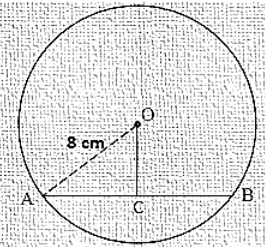Radius of circle (OA) = 8 cm

Chord (AB) = 12 cm

Draw OC⊥AB

We know that

The perpendicular from centre to chord bisects the chord

∴ AC = BC = 12/2 = 6 cm

Now in ΔOCA, by Pythagoras theorem

AC2 + OC2 = OA2

⟹ 62 + OC2 = 82

⟹ 36 + OC2 = 64

⟹ OC2 = 64-36

⟹ OC2 = 28

⟹ OC = √28

⟹ OC = 5.291 cm

Question: 2

Find the length of a chord which is at a distance of 5 cm from the centre of a circle of radius 10 cm.

Solution:

Given that,Distance (OC) = 5 cm

Radius of the circle (OA) = 10 cm

In ΔOCA, by Pythagoras theorem

OC2 + AC2 = OA2

⟹ 52 + AC2 = 102

⟹ 25 + AC2 = 100

⟹ AC2 = 100 - 25

⟹ AC2 = 75

⟹ AC = √75

⟹ AC = 8.66 cm

We know that, the perpendicular from the centre to chord bisects the chord

Therefore, AC = BC = 8.66cm

Then the chord AB = 8.66 + 8.66

= 17.32 cm

Question: 3

Find the length of a chord which is at a distance of 4 cm from the centre of a circle of radius 6 cm.

Solution:

Given that,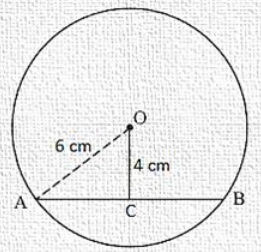Radius of the circle (OA) = 6 cm

Distance (OC) = 4 cm

In ΔOCA, by Pythagoras theorem

AC2 + OC2 = OA2

⟹ AC2 + 42 = 62

⟹ AC2 = 36 - 16

⟹ AC2 = 20

⟹AC = √20

⟹ AC = 4.47 cm

We know that the perpendicular distance from centre to chord bisects the chord.

AC = BC = 4.47 cm

Then AB = 4.47 + 4.47

= 8.94 cm

Question: 4

Two chords AB, CD of lengths 5 cm, 11 cm respectively of a circle are parallel. If the distance between AB and CD is 3 cm, find the radius of the circle.

Solution:

Construction: Draw OP⊥ CD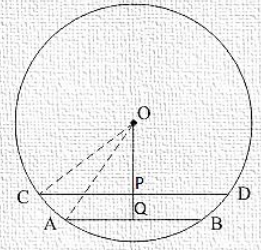Chord AB = 5 cm

Chord CD = 11cm

Distance PQ = 3 cm

Let OP = x cm

And OC = OA = r cm

We know that the perpendicular from centre to chord bisects it.

∴ CP = PD = 11/2 cm

And AQ = BQ = 5/2 cm

In ΔOCP, by Pythagoras theorem

OC2 = OP2 + CP2

⇒ r2 = x2 + (11/2)2 ... (i)

In ΔOQA, by Pythagoras theorem

OA2 = OQ2 + AQ2

⇒ r2 = (x + 3)2 + (5/2)2 ... (ii)

Compare equation (i) and (ii)

⇒ (x + 3)2 + (5/2)2 = x2 + (11/2)2

⇒ x2 + 6x + 9 + 25/4 = x2 + 121/4

⇒ x2 − x2 + 6x = 121/4 − 25/4 − 9

⇒ 6x = 15

⇒ x = 15/6 = 5/2

Question: 5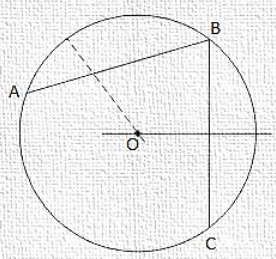Give a method to find the centre of a given circle.

Solution:

Steps of Construction:

(1) Take three points A, B and C on the given circle.

(2) Join AB and BC.

(3) Draw the perpendicular bisectors of the chord AB and BC which intersect each other at O.

(4) Point O will give the required circle because we know that, the Perpendicular bisectors of chord always pass through the centre.

Question: 6

Prove that the line joining the mid-point of a chord to the centre of the circle passes through the mid-point of the corresponding minor arc.

Solution:

Given: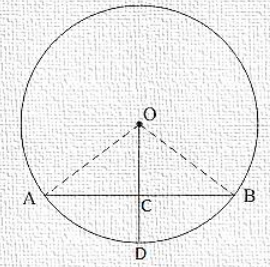C is the mid-point of chord AB.

To prove: D is the mid-point of arc AB.

Proof:

In ΔOAC and ΔOBC

OA = OB [Radius of circle]

OC = OC [Common]

AC = BC [C is the mid-point of AB]

Then ΔOAC ≅ ΔOBC [By SSS condition]

∴ ∠AOC = ∠BOCHence, D is the mid-point of arc AB.

Question: 7

Prove that a diameter of a circle which bisects a chord of the circle also bisects the angle subtended by the chord at the centre of the circle.

Solution:

Given: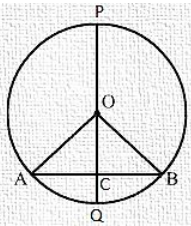PQ is a diameter of circle which bisects the chord AB at C.

To Prove: PQ bisects ∠AOB

Proof:

In ∠AOC and ∠BOC

OA = OB [Radius of circle]

OC = OC [Common]

AC = BC [Given]

Then ΔAOC ≅ ΔBOC [By SSS condition]

∠AOC = ∠BOC   [C.P.C.T]

Hence PQ bisects ∠AOB.

Question: 8

Prove that two different circles cannot intersect each other at more than two points.

Solution:

Suppose two circles intersect in three points A, B, C.

Then A, B, C are non-collinear so a unique circle passes through these three points. This is contradiction to the face that two given circles are passing through A, B, C.

Hence, two circles cannot intersect each other at more than two points.

Question: 9

A line segment AB is of length 5 cm. Draw a circle of radius 4 cm passing through A and B. Can you draw a circle of radius 2 cm passing through A and B? Give reason in support of your answer.

Solution:

(1) Draw a line segment AB of 5cm.

(2) Draw the perpendicular bisectors of AB.

(3) With centre A and radius of 4cm, draw an arc which intersects the perpendicular bisector at point O. The point O will be the required centre.

(4) Join OA.

(5) With centre O and radius OA, draw a circle.

No, we cannot draw a circle of radius 2 cm passing through A and B because when we draw an arc of radius 2 cm with centre A, the arc will not intersect the perpendicular bisector and we will not find the centre.

Question: 10

An equilateral triangle of side 9 cm is inscribed in a circle. Find the radius of the circle.

Solution:

Let ABC be an equilateral triangle of side 9 cm and let AD is one of its median.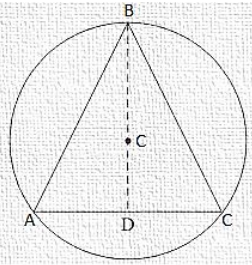Let G be the centroid of ΔABC. Then AG: GD = 2 : 1

We know that in an equilateral triangle, centroid coincides with the circum centre.

Therefore, G is the centre of the circumference with circum radius GA.

Also G is the centre and GD is perpendicular to BC.

Therefore, In right triangle ADB, we have

⟹ 92 = AD2 + DB2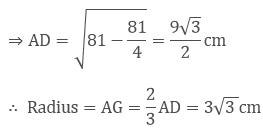Question: 11

Given an arc of a circle, complete the circle.

Solution: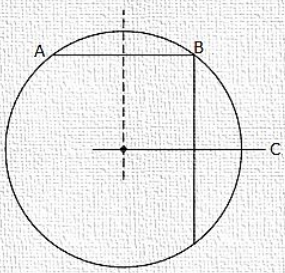Steps of Construction:

(1) Take three points A, B and C on the given arc.

(2) Join AB and BC.

(3) Draw the perpendicular bisectors of chords AB and BC which intersect each other at point O. Then O will be the required centre of the required circle.

(4) Join OA.

(5) With centre O and radius OA, complete the circle.

Question: 12

Draw different pairs of circles. How many points does each pair have in common? What is the maximum number of common points?

Solution:

Each pair of circles have 0, 1 or 2 points in common.

The maximum number of points in common is ‘2’.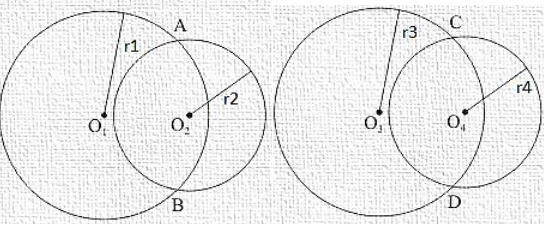Question: 13Suppose you are given a circle. Give a construction to find its centre.

Solution:

Steps of Construction:

(1) Take three points A, B and C on the given circle.

(2) Join AB and BC.

(3) Draw the perpendicular bisectors of chord AB and BC which intersect each other at O.

(4) Point O will be the required centre of the circle because we know that the perpendicular bisector of the chord always passes through the centre.

Question: 14

Two chords AB and CD of lengths 5 cm and 11 cm respectively of a circle are parallel to each other and are opposite side of its centre. If the distance between AB and CD is 6 cm, find the radius of the circle.

Solution:

Draw OM⊥AB and ON⊥CD.Join OB and OD.

BM = AB/2 = 5/2 [Perpendicular from the centre bisects the chord]

ND = CD/2 = 11/2

Let ON be x, so OM will be 6 - x.

ΔMOB

OM2 + MB2 = OB2

(6 − x)2 + (5/2)2 = OB2

36 + x2 − 12x + 25/4 = OB2 ... (i)

In ΔNOD

ON2 + ND2 = OD2

x2 + (11/2)2 = OD2

x2 + 121/2 = OD2.... (ii)

We have OB = OD. [Radii of same circle]

So, from equation (i) and (ii).

36 + x2 − 12x + 25/4 = x2 + 121/2

⇒ 12x = 36 + 25/4 − 121/4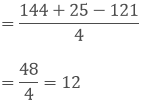x = 1

From equation (ii)

(1)2 + (121/4) = OD2

OD2 = 1 + 121/4 = 125/4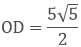So, the radius of the circle is found to be 55√2 cm

Question: 15

The lengths of two parallel chords of a circle are 6 cm and 8 cm. If the smaller chord is at a distance of 4 cm from the centre, what is the distance of the other chord from the centre?

Solution:

Distance of smaller chord AB from centre of circle = 4 cm, OM = 4 cm

MB = AB/2 = 6/2 = 3 cm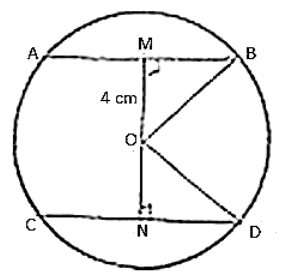In ΔOMB

OM2 + MB2 = OB2

42 + 92 = OB2

16 + 9 = OB2

OB = √25

OB = 5 cm

In ΔOND

OD = OB = 5 cm [Radii of same circle]

ND = CD/2 = 8/2 = 4 cm

ON2 + ND2 = OD2

ON2 + 42 = 52

ON2 = 25 − 16

ON = √9

ON = 3 cm

So, the distance of bigger chord from the circle is 3 cm.
```### Course Features

• 728 Video Lectures
• Revision Notes
• Previous Year Papers
• Mind Map
• Study Planner
• NCERT Solutions
• Discussion Forum
• Test paper with Video Solution